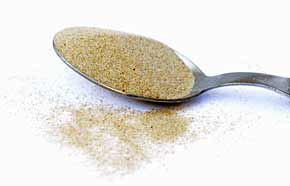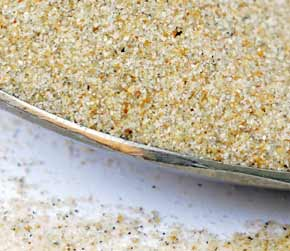﻿ Beach Sand 1 cubic millimeter volume to cups Metric converter

# beach sand conversion

## Amount: 1 cubic millimeter (mm3) of volume Equals: 0.0000040 cups Metric (cup) in volume

Converting cubic millimeter to cups Metric value in the beach sand units scale.

TOGGLE :   from cups Metric into cubic millimeters in the other way around.

## beach sand from cubic millimeter to cup Metric Conversion Results:

### Enter a New cubic millimeter Amount of beach sand to Convert From

* Whole numbers, decimals or fractions (ie: 6, 5.33, 17 3/8)
* Precision is how many numbers after decimal point (1 - 9)

Enter Amount :
Decimal Precision :

CONVERT :   between other beach sand measuring units - complete list.

Conversion calculator for webmasters.

## Beach sand weight vs. volume units

Beach sand has quite high density, it's heavy and it easily leaks into even tiny gaps or other opened spaces. No wonder it absorbs and conducts heat energy from the sun so well. However, this sand does not have the heat conductivity as high as glass does, or fireclay and firebricks, or dense concrete. A fine beach sand in dry form was used for taking these measurements.Convert beach sand measuring units between cubic millimeter (mm3) and cups Metric (cup) but in the other reverse direction from cups Metric into cubic millimeters.

 conversion result for beach sand: From Symbol Equals Result To Symbol 1 cubic millimeter mm3 = 0.0000040 cups Metric cup

# Converter type: beach sand measurements

This online beach sand from mm3 into cup converter is a handy tool not just for certified or experienced professionals.

First unit: cubic millimeter (mm3) is used for measuring volume.
Second: cup Metric (cup) is unit of volume.

## beach sand per 0.0000040 cup is equivalent to 1 what?

The cups Metric amount 0.0000040 cup converts into 1 mm3, one cubic millimeter. It is the EQUAL beach sand volume value of 1 cubic millimeter but in the cups Metric volume unit alternative.

How to convert 2 cubic millimeters (mm3) of beach sand into cups Metric (cup)? Is there a calculation formula?

First divide the two units variables. Then multiply the result by 2 - for example:
4.0E-6 * 2 (or divide it by / 0.5)

QUESTION:
1 mm3 of beach sand = ? cup

1 mm3 = 0.0000040 cup of beach sand

## Other applications for beach sand units calculator ...

With the above mentioned two-units calculating service it provides, this beach sand converter proved to be useful also as an online tool for:
1. practicing cubic millimeters and cups Metric of beach sand ( mm3 vs. cup ) measuring values exchange.
2. beach sand amounts conversion factors - between numerous unit pairs variations.
3. working with mass density - how heavy is a volume of beach sand - values and properties.

International unit symbols for these two beach sand measurements are:

Abbreviation or prefix ( abbr. short brevis ), unit symbol, for cubic millimeter is:
mm3
Abbreviation or prefix ( abbr. ) brevis - short unit symbol for cup Metric is:
cup

### One cubic millimeter of beach sand converted to cup Metric equals to 0.0000040 cup

How many cups Metric of beach sand are in 1 cubic millimeter? The answer is: The change of 1 mm3 ( cubic millimeter ) volume unit of beach sand measure equals = to volume 0.0000040 cup ( cup Metric ) as the equivalent measure within the same beach sand substance type.

In principle with any measuring task, switched on professional people always ensure, and their success depends on, they get the most precise conversion results everywhere and every-time. Not only whenever possible, it's always so. Often having only a good idea ( or more ideas ) might not be perfect nor good enough solution. If there is an exact known measure in mm3 - cubic millimeters for beach sand amount, the rule is that the cubic millimeter number gets converted into cup - cups Metric or any other beach sand unit absolutely exactly.

Conversion for how many cups Metric ( cup ) of beach sand are contained in a cubic millimeter ( 1 mm3 ). Or, how much in cups Metric of beach sand is in 1 cubic millimeter? To link to this beach sand cubic millimeter to cups Metric online converter simply cut and paste the following.
The link to this tool will appear as: beach sand from cubic millimeter (mm3) to cups Metric (cup) conversion.

I've done my best to build this site for you- Please send feedback to let me know how you enjoyed visiting.